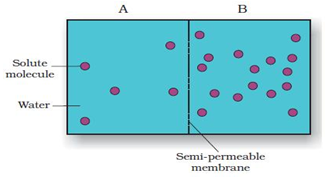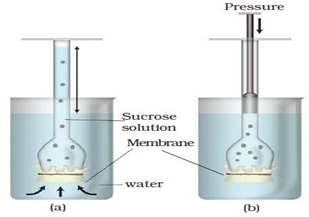Apply concept of water potential and osmosis

Which chamber has lower solute potential at equilibrium?

1. Chamber A
2. Chamber B
3. Both will have equal values
4. Cannot be predicted
High Yielding Test Series + Question Bank - NEET 2020

Difficulty Level:Apply concept of water potential and osmosis

If B chamber has water potential of -2000 kPa and the A has water potential of -1000kPa, which is the chamber that has the highest Water potential?

1. Chamber A
2. Chamber B
3. Both will have equal values
4. Cannot be predicted
High Yielding Test Series + Question Bank - NEET 2020

Difficulty Level:Apply concept of water potential and osmosis

If two solutions, A with water potential 0.2 MPa and B with water potential 0.1 MPa are separated by a selectively permeable membrane, then what will be the direction of movement of water?

1. From A to B
2. From B to A
3. No net osmosis
4. Cannot be predicted
High Yielding Test Series + Question Bank - NEET 2020

Difficulty Level:

Apply concept of water potential and osmosisThe pressure applied in this experiment is

1. Hydrostatic Pressure
2. Colloidal pressure
3. Osmotic pressure
4. Pressure potential
High Yielding Test Series + Question Bank - NEET 2020

Difficulty Level:

Osmotic pressure is a function of

1. Osmotic potential
2. Solute concentration
3. Solvent amount
4. Water potential
High Yielding Test Series + Question Bank - NEET 2020

Difficulty Level:

Osmotic pressure is the

1. Pressure required to stop the water diffusing in
2. Function of solute concentration
3. More the solute, more is the pressure needed to stop endosmosis
4. All of these
High Yielding Test Series + Question Bank - NEET 2020

Difficulty Level:

Numerically osmotic pressure is equal to  the

1. Osmotic potential
2. Solute potential
3. Both A and B but sign is opposite
4. Pressure potential
High Yielding Test Series + Question Bank - NEET 2020

Difficulty Level:

Isotonic solution is

1. A solution which is more concentrated than the cytoplasm
2. A solution which is more dilute than the cytoplasm
3. A solution which balances the osmotic pressure of the cytoplasm
4. A solution which is less dilute than the cytoplasm
High Yielding Test Series + Question Bank - NEET 2020

Difficulty Level:

Hypotonic solution

1. solution which is more concentrated than the cytoplasm
2. A solution which is more dilute than the cytoplasm
3. A solution which balances the osmotic pressure of the cytoplasm
4. A solution which is less dilute than the cytoplasm
High Yielding Test Series + Question Bank - NEET 2020

Difficulty Level:

Hypertonic solution

1. solution which is more concentrated than the cytoplasm
2. A solution which is more dilute than the cytoplasm
3. A solution which balances the osmotic pressure of the cytoplasm
4. A solution which is equal to that of cytoplasm in terms of concentration